# Rotation surface

surface of rotation, rotational surface

A surface generated by the rotation of a plane curvearound an axis in its plane. Ifis defined by the equations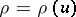,, the position vector of the surface of rotation is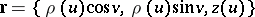, whereis the parameter of the curve,is the distance between a point on the surface and the axisof rotation andis the angle of rotation. The line element of the surface of rotation is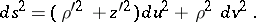The Gaussian curvature is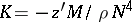, the mean curvature is, where,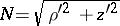. The lines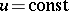are called parallels of the surface of rotation and are circles located in a plane normal to the axis of rotation, with their centres on this axis. The linesare called meridians; they are all congruent to the rotating curve and lie in planes passing through the axis of rotation. The meridians and the parallels of a surface of rotation are its curvature lines and form an isothermal net.

A surface of rotation allows for a deformation into another surface of rotation, under which its net of curvature lines is preserved and therefore is a principal base of the deformation. The umbilical points (cf. Umbilical point) of a surface of rotation are characterized by the property that the centre of curvature of the meridian lies on the axis of rotation. The product of the radius of a parallel by the cosine of the angle of intersection of the surface of rotation with the parallel is constant along a geodesic (Clairaut's theorem).

The only minimal surface of rotation is the catenoid. A ruled surface of rotation is a one-sheet hyperboloid or one of its degeneracies: a cylinder, a cone or a plane. A surface of rotation with more than one axis of rotation is a sphere or a plane.

The metric of a surface of rotation can be presented in the form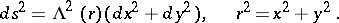(1)

For the existence of metrics of the form (1) and for isometric immersions of these inas surfaces of rotation see .